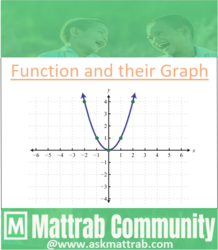Algebraic Functions and their Graphs﻿1. Linear Function
→ It is the function in the form : y = m x + c
The graph of such functions is a straight line.
→ If m(slope) is positive then the line rises to the right and if m is negative then the line falls to the right.
Graph→ The dotted lines shown in the graph represents linear function.

﻿
2. Constant Function
→
﻿It is the function in which all its functional value are same.(i.e., if the range of the function is singleton.)
→  The graph of a constant function is a horizontal line parallel to x-axis.
Graph→ The dotted lines shown in the graph represents constant function.

→ It is the function in the form: ax2 + bx + c
The graph of quadratic function gives a parabola.
Graph→The dotted parabola shown in the graph represents a quadratic equation.

→ It is the function in the form: ax3 + bx2 + cx + d
→
The graph of quadratic function gives a trivalent graph.
Graph→The dotted trivalent graph(not necessarily to pass through origin) as shown above represents cubic function.

﻿5. Logarithm Function and Exponential Function
→ Logarithm function is the function in the form: y= logax
→Exponential function is the function in the: y = ax .
→Logarithm Function and Exponential function are inverse to each other.
→ The graph of function
y= logax is equivalent to x=ay and the inverse of y= logax gives the graph of exponential function y=ax .
→ Both the functions give arc in the graph

Graph→Here, the functions representing the dotted and solid arc are inverse to each other. In this case, if solid arc represents the exponential function then dotted arc represents logarithm function and vice versa.

Note:

Polynomial function are the function in the form anxn + an−1xn−1 + ....... + a2x2 ﻿+ a1x + a0 ﻿The constant function, linear function, quadratic function, cubic function are some examples of polynomial functions. There is no specific function for polynomial function as a whole so it's examples are studied separately. The graph of polynomial function maybe continuous or broken.